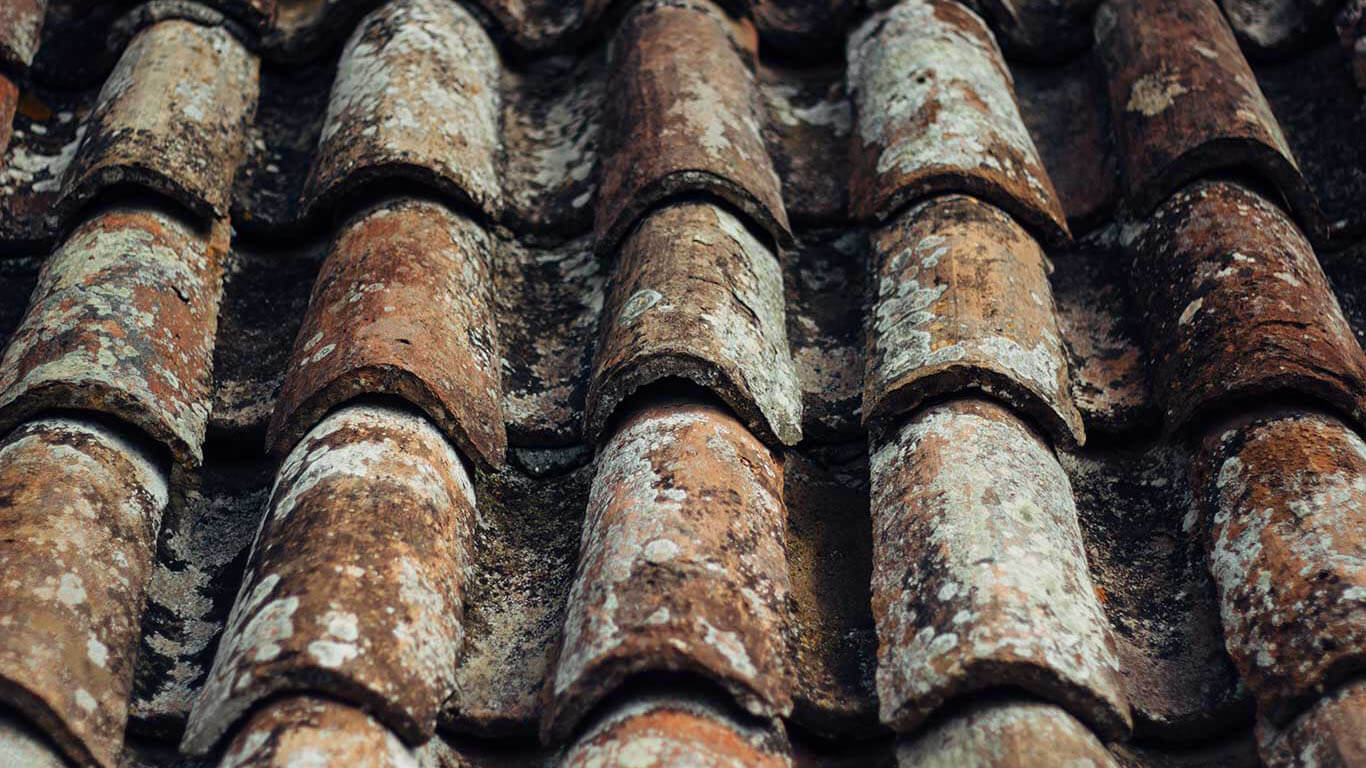Roofing is measured in squares. Roofing contractors can use a simple geometry and online calculators to figure out a roof area and buy materials. The key is to be as accurate as possible with your measurements and calculations since falling short, or buying more material than you need, could mean thousands of dollars might get added to your budget.

Step 1: This step entails making a rough sketch of the roof shape so you can clearly record your readings. This will make it easier to mark measurements and estimate how much material you actually need to finish the project successfully. For this example we will break this roof up into sections labelled A through E.

Step 2: Area of a Triangle
The area of a triangle is the space contained within its 3 sides. To find out the area of a triangle, we need to know the length of its three sides. The sides should be measured in feet (ft) for square footage calculations and if needed, converted to inches (in), yards (yd), centimetres (cm), millimetres (mm) and metres (m).

#### Formula:

Area of a Triangle = (1/4) x √ [ (a+b+c) x (b+c-a) x (c+a-b) x (a+b-c) ]
Answer = ((1/4) x √ [ (a+b+c) x (b+c-a) x (c+a-b) x (a+b-c) ])
Abbreviations of unit area: ft2, in2, yd2, cm2, mm2, m2

A conversion chart helps for square footage calculations. This is why we have provided charts for converting between different units of measurement. Whether it is the area of a rectangle, square or the area of a triangle you can utilize our calculators and figure out how much border material you need.

Step 3: There are some rectangular areas as well. You can utilize our calculator here as well.

Area of A Rectangle
The area of a square is the space contained within a set of parallel lines – each set of different lengths. These lines should be measured in feet (ft) for square footage calculations and if needed, converted to inches (in), yards (yd), centimetres (cm), millimetres (mm) and metres (m).

#### Formula:

Area of a Square= Side length x Side width
Answer = (Length x Width) square area
Abbreviations of unit area: ft2, in2, yd2, cm2, mm2, m2We can now use the same calculation method and apply it to a more complex roofing system. This is closer to a real-world scenario and needs to be examined closely for roofing novices to be able to accurately measure a roof. In the overhead drawing below you can see both a hip-end section, and gable ends. It may seem like a daunting task at first but it can be made easier if done in sections.

If you want to DIY this, we have some advice for you from The Family Handyman Magazine: “Before you embark on the roofing process though – remember roofing is hard work. There’s no hiding from the elements. You can’t be afraid of heights and you need to be pretty fit. Before committing to this project, try this: Get out a ladder and climb up onto your roof. If you can’t walk around on it comfortably, hire a pro. If you passed this first test, go to the lumberyard or home centre and throw a bundle of shingles onto your shoulder. Imagine yourself carrying that load up a ladder many, many times.”

Still up for it? Then go for it! Remember, keep building and leave the calculation part to us! Want more calculators? Let us know which calculators you need and we will try our best to add them to our website.

Want us to cover a specific topic? Write to us.